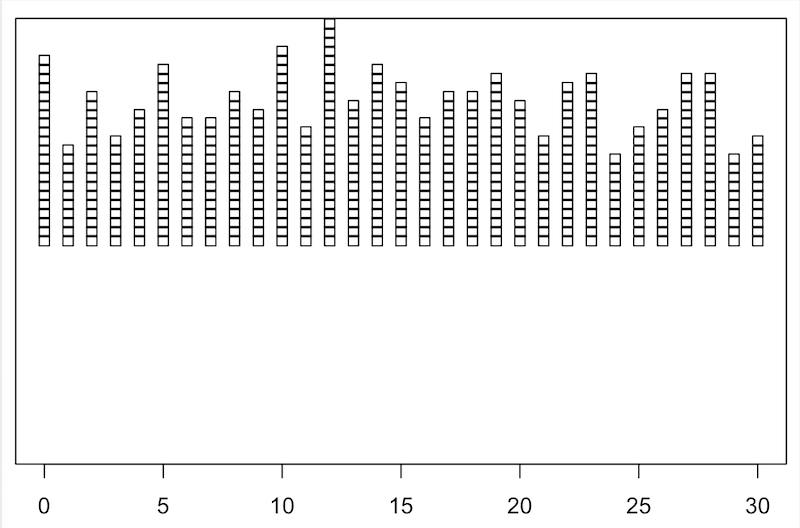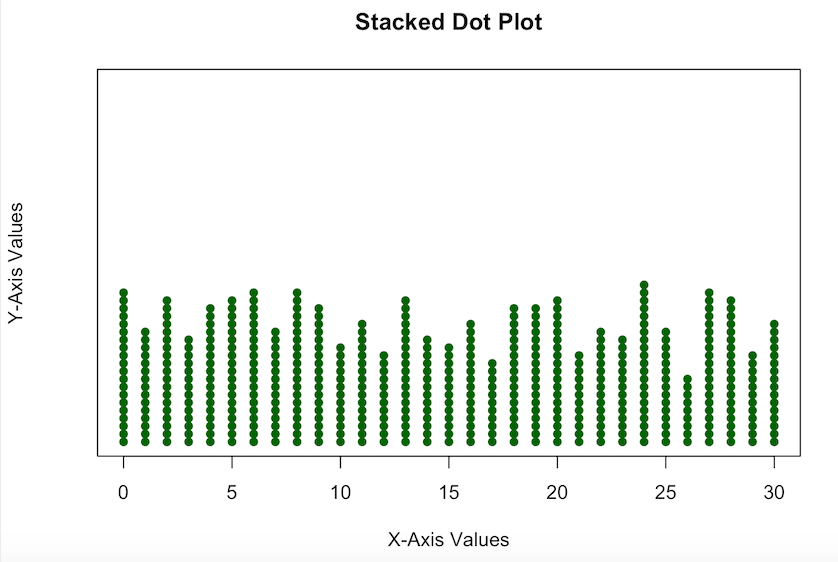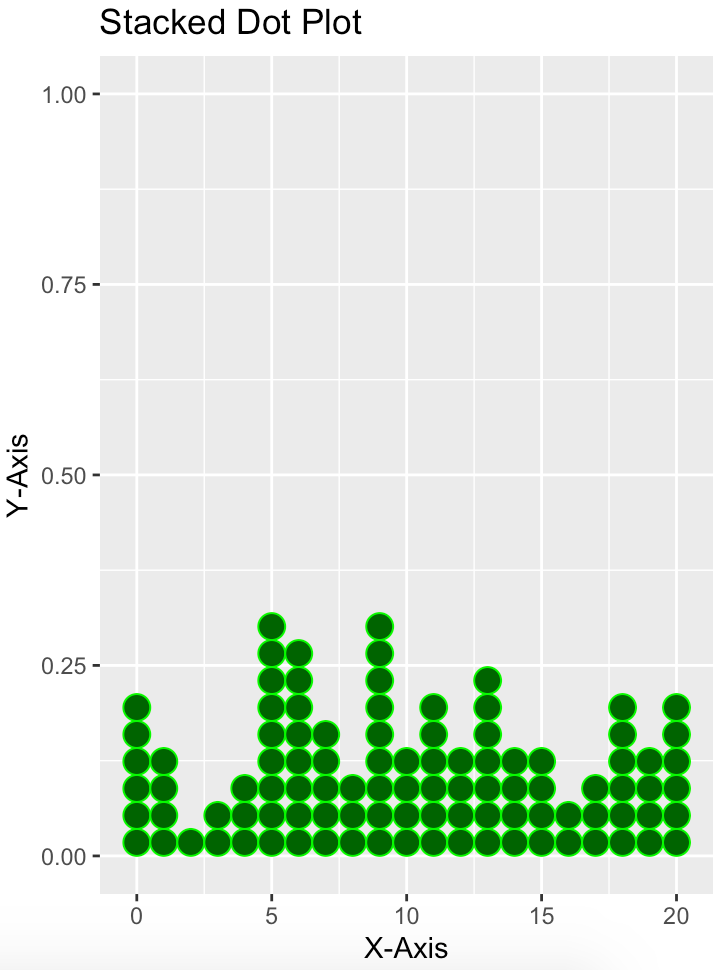Related Articles
How to Create a Stacked Dot Plot in R ?
• Difficulty Level : Medium
• Last Updated : 28 Apr, 2021

A stacked dotplot is a type of plot that displays frequencies using dots, piled one over the other. Mainly 2 methods are there, to make a stacked dot plot and both of them are discussed in this article.

### Method 1: Using stripchart()

So, using the first method, stripchart method, to create our stacked dot plot. For instance, if we don’t have a set of values, we can even make them, serve our purpose. We would create a set of values that would contain numbers ranging from 0 to 30, including both the ranges too, i.e., the set of values would also include 0 and 30. After creating the set of values, we would plot our stacked dotplot based on those values. We would use the function set.seed(), to reproduce a particular sequence of ‘random’ numbers. stripchart produces one-dimensional scatter plots (or dot plots) of the given data.

Syntax: stripchart(x, …)

Example:

## R

 `# sets the starting number used ``# to generate a sequence of random ``# numbers``set.seed``(0)`` ` `# shows 100 such randomly generated``# numbers from 0 to 20``data <- ``sample``(0:30, 500, replace = ``TRUE``)`` ` `# creates the stacked data plot``stripchart``(data, method = ``"stack"``)`

Output:But, the dot plot which we made, is not so pleasing, like the whole stacked dotplot is somewhat above the X-axis, so now we are going to edit it a bit, to make it look more interesting.

Example:

## R

 `# sets the starting number which is used``# to generate a sequence of random numbers``set.seed``(0)`` ` `# shows 100 such randomly generated ``# numbers from 0 to 20``data <- ``sample``(0:30, 500, replace = ``TRUE``)`` ` `# creats the stacked dotplot, given ``# some more parameters to make the``# stacked dotplot look more attractive``stripchart``(data, method = ``"stack"``, at = 0, ``           ``pch = 16, col = ``"darkgreen"``,``           ``main = ``"Stacked Dot Plot"``, ``           ``xlab = ``"X-Axis Values"``, ``           ``ylab = ``"Y-Axis Values"``)`

Output:### Method 2: Using geom_dotplot()

In a dot plot, the width of a dot corresponds to the bin width (or maximum width, depending on the binning algorithm), and dots are stacked, with each dot representing one observation.

Syntax: geom_dotplot()

Parameter:

• dotsize:  The diameter of the dots relative to binwidth, default 1.
• stackratio: how close to stack the dots. Default is 1, where dots just touch. Use smaller values for closer, overlapping dots.
• fill: interior colour of the dots in the stack.
• color: exterior outline colour of the dots in the stack

Example:

## R

 `# loads required package``require``(ggplot2)`` ` `# sets the starting number used ``# to generate a sequence of random ``# numbers``set.seed``(0)`` ` `# shows 100 such randomly generated``# numbers from 10 to 50``data <- ``data.frame``(x = ``sample``(10:50, 100, replace = ``TRUE``))`` ` `# creates the stacked dot plot``ggplot``(data, ``aes``(x = x)) + ``geom_dotplot``()`

Output:Again, for this one too, we can make it a bit more interesting, adding some more parameters.

Example:

## R

 `# load ggplot2``library``(ggplot2)`` ` `set.seed``(0)``data <- ``data.frame``(x = ``sample``(0:20, 100, replace = ``TRUE``))`` ` `# create customized stacked dot plot``ggplot``(data, ``aes``(x = x)) +``  ``geom_dotplot``(dotsize = 1.5, stackratio = 1, ``               ``fill = ``"darkgreen"``, color = ``"green"``) + ``labs``(title = ``"Stacked Dot Plot"``, x = ``"X-Axis"``, y = ``"Y-Axis"``)`

Output:My Personal Notes arrow_drop_up